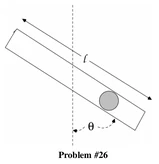162 Pages

## ProblemEditA particle of mass m is placed in a smooth uniform tube of mass M and length l.  The tube is free to rotate about its center in a vertical plane.  The system is started from rest with the tube horizontal and the particle a distance $r_0$ from the center of the tube.

For what length of the tube will the particle leave the tube when $\dot{\theta}=\omega$ is a maximum and $\theta=\theta_m$?  Your answer should be in terms of $\omega$ and $\theta_m$.

## SolutionEdit

Find the Lagrangian and Euler-Langrange equation of motion
$T=\frac{1}{2}m\dot{r}^2+\frac{1}{2}mr^2\dot{\theta}^2+\frac{1}{2}I\dot{\theta}^2$ (where $I=\frac{1}{12}M l^2$ is the moment of inertia of the tube about its center of mass).

$V=-mg\frac{l}{2}\cos{\theta}$

$L=\frac{1}{2}m\dot{r}^2+\frac{1}{2}mr^2\dot{\theta}^2+\frac{1}{2}I\dot{\theta}^2 +mg\frac{l}{2}\cos{\theta}$

so
$\ddot{\theta}=\frac{-1}{mr^2+I}\left(2mr\dot{r}\dot{\theta}+mg\frac{l}{2}\sin{\theta}\right)$

At the moment when $\dot{\theta}$ is at a maximum:
$\ddot{\theta}=0$
$r=l/2$
$\dot{\theta}=\omega$
$\theta=\theta_m$

so

$\dot{r}_m\omega=\frac{-g}{2}\sin{\theta_m}$

or

$\dot{r}_m^2=\frac{g^2}{4\omega^2}\sin^2{\theta_m}$

where $\dot{r}_m$ is the radial component of velocity when $\theta=\theta_m$. $\dot{r}_m$ can be found by conservation of energy, namely

$E_o=0=E_f$

$E_f=\frac{1}{2}m\dot{r}_m^2+\frac{1}{2}mr_{m}^2\omega^2+\frac{1}{2}I\omega^2-mgr_m\cos{\theta_m}=0$

subbing $r_m=\frac{l}{2}$

$\dot{r}_m^2=gl\cos{\theta_m}-\left(\frac{l}{2}\right)^2\omega^2-\frac{I\omega^2}{m}$

Therefore,

$\frac{g^2}{4\omega^2}\sin^2{\theta_m}=gl\cos{\theta_m}-\left(\frac{l}{2}\right)^2\omega^2-\frac{I\omega^2}{m}$

or

$\left(\frac{3m+M}{3m}\right)\omega^2l^2-(4gl\cos{\theta_m})+\frac{g^2}{\omega^2}\sin^2{\theta_m}=0$

which is a quadratic equation in $l$, which can be solved for in terms of g, m, M, $\omega$, and $\theta_m$

Community content is available under CC-BY-SA unless otherwise noted.